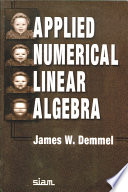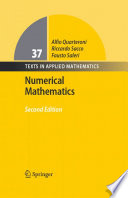### Numerical Linear Algebra

#### Course Description

Diagonalization of a matrix. Jordan form. Positively definite matrices and quadratic forms.Matrix norms. QR and SVD decomposition of the matrix and applications. Iterative methods for solving linear systems. Approximations of eigenvalues.

#### Learning Outcomes

1. define and describe fundamental concepts such as matrix norms, singular and eigen values and vectors
2. recognize types of matrices, such as ortogonal, unitary, symetric, Hermitian, normal and positive definite matrices
3. describe Jordan and Schure form of the matrix and a notion of a diagonalizable matrix
4. apply matrix transformation in order to transform a matrix into triangular, Hessenberg and tridiagonal form
5. derive and utilize SVD and QR factorization of the matrix for efficiently solving problems in practice
6. analyze and employ iterative methods for linear algebraic systems
7. explain and employ iterative algorithms for computing eigenvalues
8. Relate the quality of obtained numerical solution to derived theoretical results

#### Forms of Teaching

Lectures

Lectures are held in two cycles, 3 hours per week

Partial e-learning

Students will solve online quizzes

Laboratory

During the semester students will work on program tasks

Continuous Assessment Exam
Type Threshold Percent of Grade Threshold Percent of Grade
Laboratory Exercises 0 % 10 % 0 % 10 %
Seminar/Project 0 % 10 % 0 % 10 %
Mid Term Exam: Written 0 % 40 % 0 %
Final Exam: Written 0 % 40 %
Exam: Written 0 % 80 %

#### Week by Week Schedule

1. Symmetric and i Hermitian (self-adjoint) matrices; Gramm-Schmidt's orthogonalization; Orthogonal and unitary matrices; Diagonalization of a matrix
2. Spectral theorem for symmetric matrices, Nilpotent matrices; Jordan cells; Minimal polynomial
3. Positively (negatively) semidefinite matrices; Positively (negatively) definite matrices; Indefinite matrices; Quadratic forms and their diagonalization; Definite and indefinite quadratic forms; Signature; Minimum/maximum and saddle points of a quadratic form
4. Operator norms; The space L(X,Y); Matrix norm; Convergence of matrices; Series of matrices, Neumann series; Spectral radius and spectral norm
5. Projectors; QR factorization, HouseHolder triangularization and its stability
6. Singular Value Decomposition and its application, Algorithms for the SVD
7. Jacobi, Gauss-Seidel and Relaxation Methods, Convergence Results for Jacobi, Gauss-Seidel and Relaxation Methods
8. Midterm exam
9. Symmetric Form of the Gauss-Seidel and SOR Methods; Implementation Issues
10. Conjugate Gradient Method and other Krylov Subspace Iterations; Finite Difference Analysis
11. Geometrical Location of the Eigenvalues, Stability and Conditioning Analysis, The Power Method; Inverse Iteration
12. The QR Method, Hessenberg Reduction; Tridiagonal and Bidiagonal Reductions, QR Iterations with Implicit Shifts
13. Methods for Eigenvalues of Symmetric Matrices (The Jacobi's Method; Tridiagonal QR Iteration, Rayleigh Quotient Iteration)
14. Applications: Analysis of an Electric Network; Problem of Beam Bending; Free Dynamic Vibration of a Bridge
15. Final exam

#### Study Programmes

[FER3-EN] Computing - study
Elective Courses (6. semester)
[FER3-EN] Electrical Engineering and Information Technology - study
Elective Courses (6. semester)

#### Literature

A. Aglić Aljinović, N. Elezović, D. Žubrinić (2017.), Linearna algebra, Element d.o.o.
Josipa Pina Milišić, Ana Žgaljić Keko (2014.), Uvod u numeričku matematiku za inženjere, Element d.o.o.N. Truhar (2010.), Numerička linearna algebra, Sveučilište J.J. Strossmayera, Odjel za matematiku
G. H. Golub; C. F. Van Loan (2013.), Matrix Computations, 4rd Edition, John Hopkins University Press
L.N. Trefethen, D. Bau (1997.), Numerical Linear Algebra, SIAMJames W. Demmel (1997.), Applied Numerical Linear Algebra, SIAMA. Quarteroni, R. Sacco, F. Saleri (2000.), Numerical Mathematics, Springer
Z. Drmač (2020.), Numerička analiza, PMF-MO (skripta)

#### General

ID 223363
Summer semester
5 ECTS
L0 English Level
L2 e-Learning
45 Lectures
0 Seminar
0 Exercises
13 Laboratory exercises
0 Project laboratory